# Hardest colleges to get into in California

Top 10 colleges in California with the lowest acceptance rates
What are the hardest colleges to get into in California? We've got you covered. We've compiled a national college database and have created a list of the hardest universities to get into in California below. These are the hardest 4 year colleges to get into in California, and sometimes some of the hardest colleges to get into in the US. You could even say these are the best colleges in California. We also include each college's average SAT and ACT scores so that you can see where you're most competitive. Read on to find out more.

## Stanford University acceptance rate

Stanford University acceptance rate is 4.3%.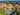The average SAT score for Stanford University is 1505.

• SAT composite: 1505
• SAT math: 770

The average ACT score for Stanford University is 34.

## California Institute of Technology acceptance rate

California Institute of Technology acceptance rate is 6.4%.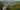The average SAT score for California Institute of Technology is 1545.

• SAT composite: 1545
• SAT math: 795

The average ACT score for California Institute of Technology is 36.

## Pomona College acceptance rate

Pomona College acceptance rate is 7.4%.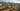The average SAT score for Pomona College is 1465.

• SAT composite: 1465
• SAT math: 745

The average ACT score for Pomona College is 34.

## Claremont McKenna College acceptance rate

Claremont McKenna College acceptance rate is 9.3%.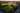The average SAT score for Claremont McKenna College is 1427.

• SAT composite: 1427
• SAT math: 730

The average ACT score for Claremont McKenna College is 32.

## University of California Los Angeles acceptance rate

University of California Los Angeles acceptance rate is 12.3%.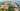The average SAT score for University of California Los Angeles is 1405.

• SAT composite: 1405
• SAT math: 715

The average ACT score for University of California Los Angeles is 31.

## University of Southern California acceptance rate

University of Southern California acceptance rate is 13%.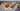The average SAT score for University of Southern California is 1440.

• SAT composite: 1440
• SAT math: 740

The average ACT score for University of Southern California is 32.

## Pitzer College acceptance rate

Pitzer College acceptance rate is 13.3%.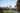The average SAT score for Pitzer College is 1410.

• SAT composite: 1410
• SAT math: 720

The average ACT score for Pitzer College is 31.

## Harvey Mudd College acceptance rate

Harvey Mudd College acceptance rate is 14.5%.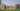The average SAT score for Harvey Mudd College is 1530.

• SAT composite: 1530
• SAT math: 790

The average ACT score for Harvey Mudd College is 35.

## Berkeley University of California acceptance rate

Berkeley University of California acceptance rate is 14.8%.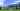The average SAT score for Berkeley University of California is 1415.

• SAT composite: 1415
• SAT math: 725

The average ACT score for Berkeley University of California is 31.

## Scripps College acceptance rate

Scripps College acceptance rate is 24.2%.The average SAT score for Scripps College is 1390.

• SAT composite: 1390
• SAT math: 690